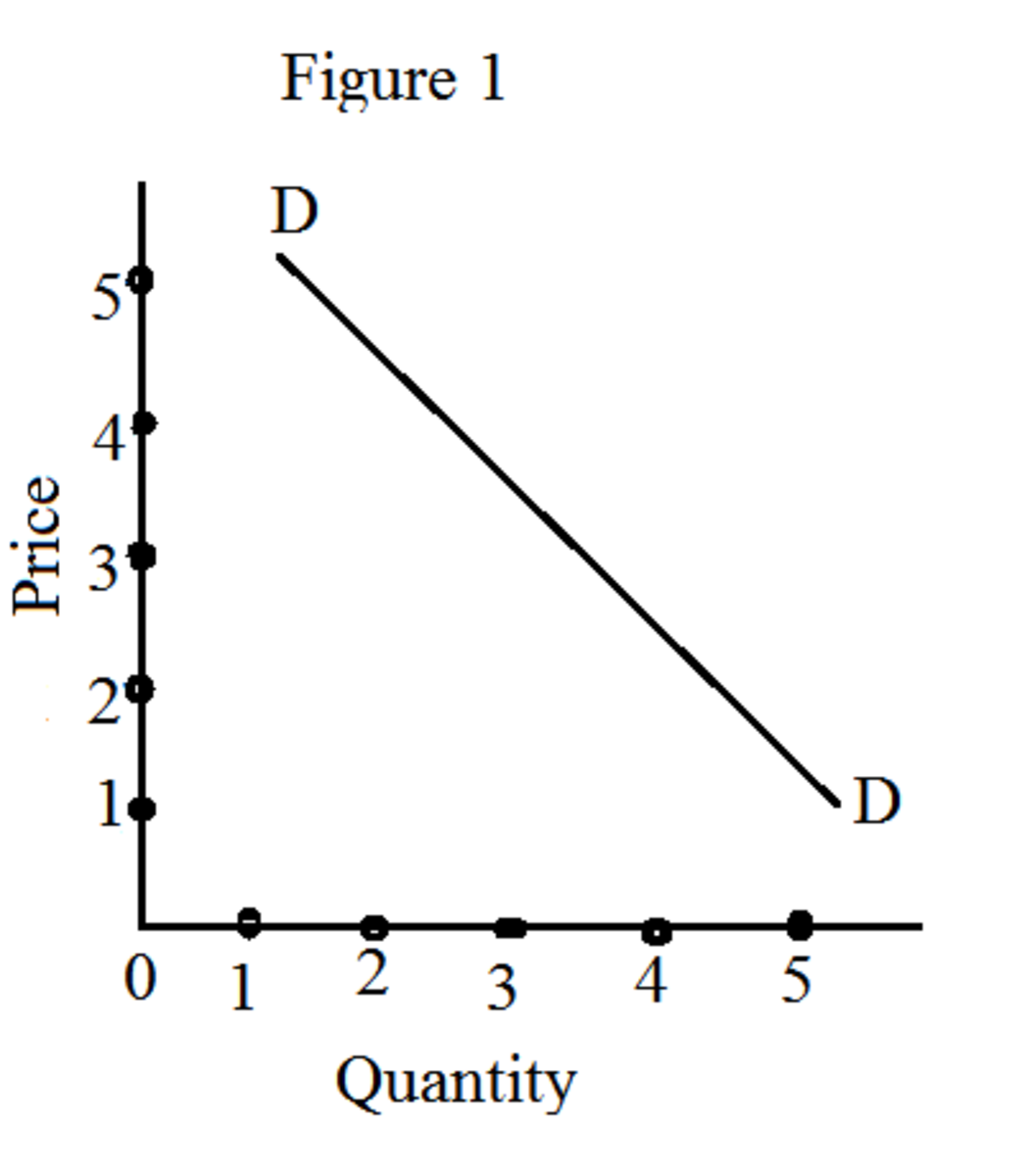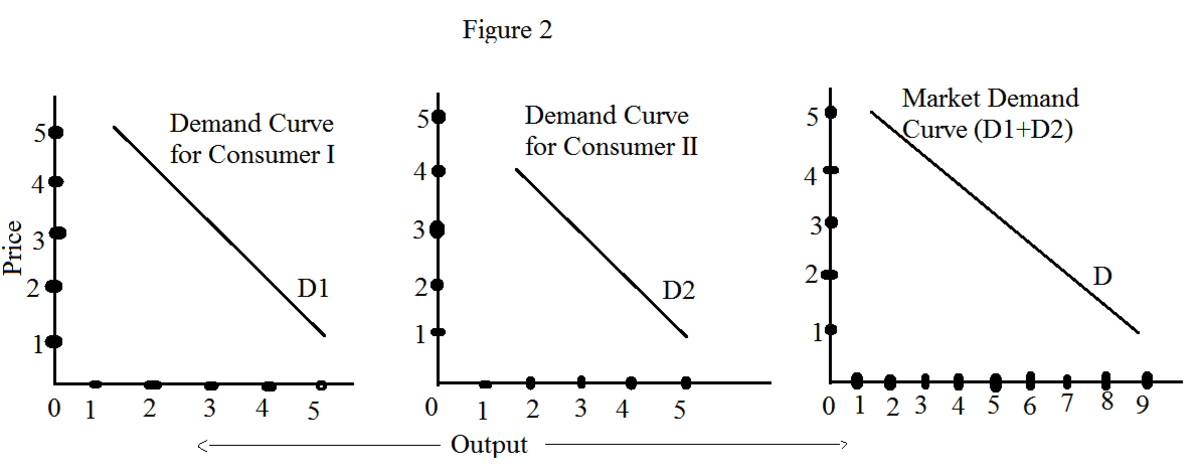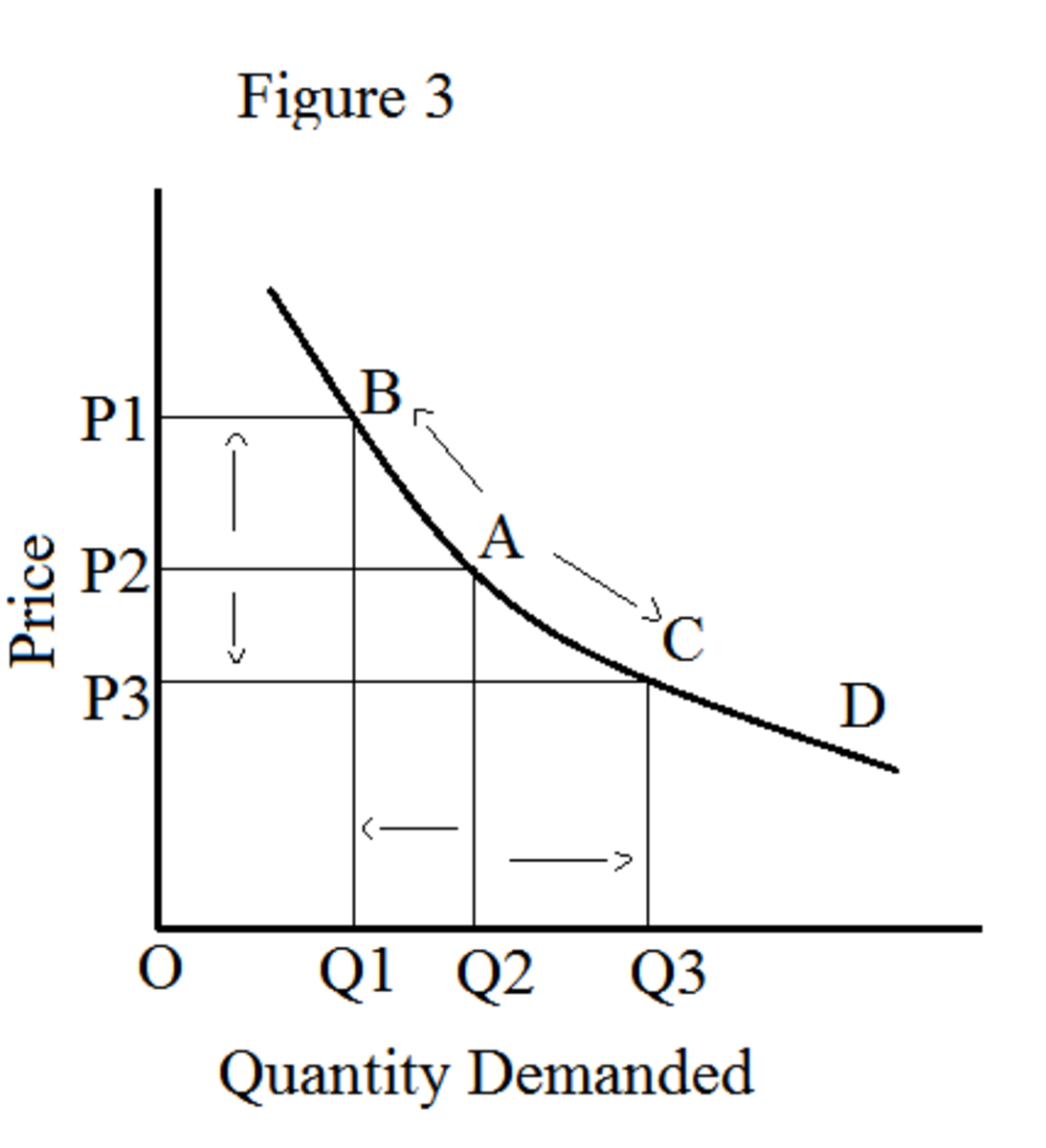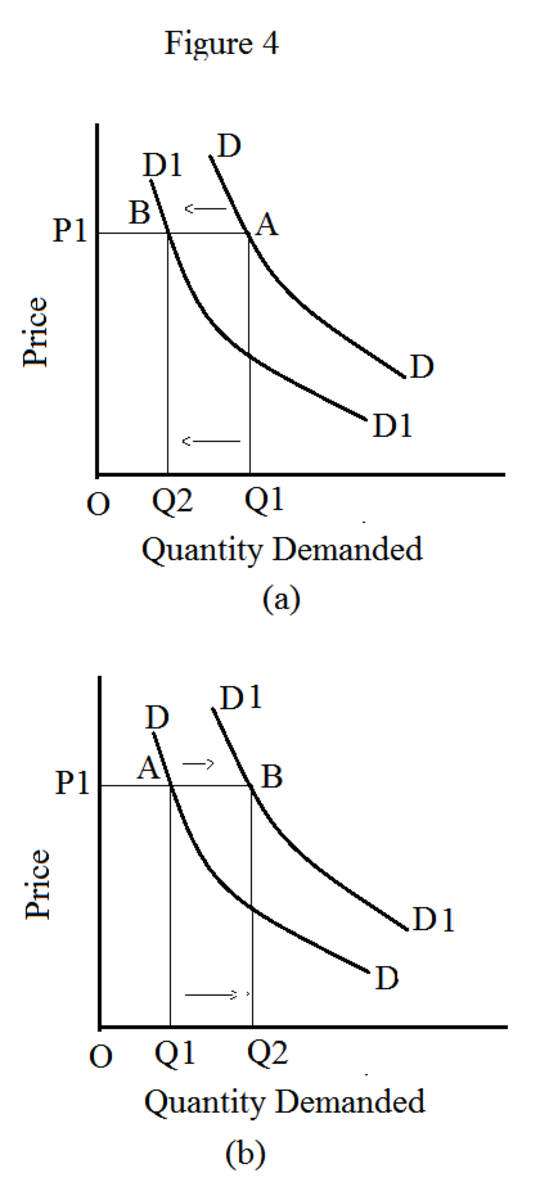Updated date:

# Demand Schedule and Demand Curve

## What is a demand schedule?

A demand schedule is crucial for constructing a demand curve. A demand schedule lists prices and corresponding quantities based on law of demand. To understand the concept of demand schedule better, let us look at the table 1.

## Table 1

Price (in \$)Quantity Demanded

5

1

4

2

3

3

2

4

1

5

Table 1 exhibits hypothetical demand schedule of an individual. In table 1, you find that there is an inverse relationship between price and quantity demanded because demand schedule follows law of demand.

## Derivation of Demand CurveAs stated earlier, you can construct a demand curve based on a demand schedule. Due to the conventional practice established by Alfred Marshall, horizontal axis measures quantity and vertical axis measures price always while deriving demand curve.

Table 1 shows that when the price is \$5, the quantity demanded is 1 unit. Similarly, when the price is \$4, the quantity demanded is 2 units and so on. As you see, there is a corresponding quantity for each price. By joining all the points established by plotting all prices and their corresponding quantities on a graph, we can obtain a demand curve. Hence, demand curve exhibits the relationship between two variables, namely price and quantity.

## Market Demand Curve

The demand schedule and demand curve showed above is the case of an individual. The analysis can be extended to a market in the same manner. A market typically consists of many customers; and every customer possesses different tastes and preferences. Hence, individual demand curves differ from person to person in their slopes and shapes. However, we are able to sum up all individual demand curves and derive a market demand curve. In other words, the market demand curve is the sum of all individual demand curves.

Illustration

For simplicity, let us assume that the market consists of only two customers. Table 2 depicts individual demand and market demand.

## Table 2: Demand Curves for two Consumers and the Market Demand Curve

Price of Commodity X (in \$)Quantity Demand by Consumer IQuantity Demanded by Consumer IIMarket Demand of X

5

1

-

1

4

2

1

3

3

3

2

5

2

4

3

7

1

5

4

9

From table 2, we understand that the market demand is obtained by summing up individual demand. The table further shows that at price \$5, the market demand is 1 unit; at price \$4, the market demand is 3 units; at price \$3, the market demand is 5 units and so on. Since there is an inverse relationship between price and quantity demanded, it is obvious that the market demand schedule also follows law of demand.

Similarly, we can derive the market demand curve (D) by the horizontal summation of individual demand curves (D1 + D2).## Movement Along the Demand Curve

Movement along the demand curve occurs when there is a change in the price. As you know, there is an inverse relationship between price and quantity demanded. Hence, reduction in the price causes a downward movement. This means that there is an increasing demand. Further, rise in the price causes an upward movement. This means that there is a decreasing demand.In figure 3, the movement from A to C represents movement along the demand curve. At point A, the price is OP2 and the quantity demanded is OQ2. When there is a movement from point A to point C, the quantity demanded increases to OQ3. Further, when there is a movement from point A to point B, the quantity demanded decreases to OQ1. Here, you can notice that the movement along the demand curve occurs because of a rise or a fall in the price level.

## Shift in the Demand Curve

There are various determinants of demand for a commodity apart from its price. A shift in the demand curve occurs when anyone of those determinants change. For example, income is one of the determinants of demand. If the household income increases, this results in an outward shift in the demand curve. This means that there is an increase in the demand.

Determinants of demand include tastes and preferences, household income, distribution of income, weather, savings pattern, expectations, consumer credit policy, real income, business cycle, taxation, subsidy, marketing techniques, population size, and value of money. As you figure out, these determinants are very closely associated with the performance of overall economy. Therefore, shift in the demand curve, which depends on these determinants exclusively, is an important concept in economics.Assume that the demand curve (DD) in figure 4(a) refers to demand for ice cream. Let us consider one of determinants of demand called weather. During rainy season, the demand for ice cream decreases. Therefore, the original demand curve (DD) shifts to the left and forms a new demand curve (D1D1). In this case, quantity demanded decreases from OQ1 to OQ2. However, the price remains unchanged at OP1.

Similarly, assume that the demand curve (DD) in figure 4(b) refers to demand for umbrella. Let us consider one of the determinants of demand called weather. During rainy season, the demand for umbrella increases. Therefore, the original demand curve (DD) shifts to the right and forms a new demand curve (D1D1). In this case, quantity demanded increases from OQ1 to OQ2. And the price remains unchanged at OP1.

To sum up, movement along the demand curve occurs because of changes in the price. Shift in the demand curve occurs because of changes in the determinants of demand other than price.

© 2013 Sundaram Ponnusamy